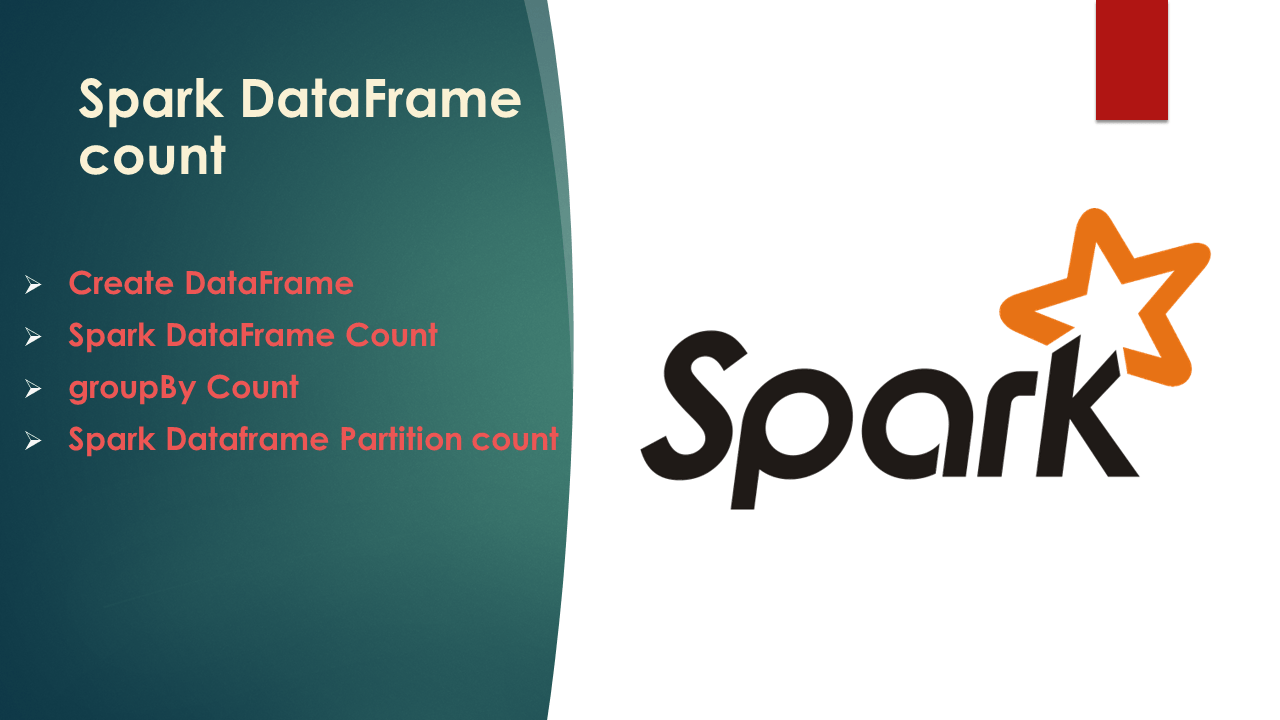# Spark DataFrame count

The spark.sql.DataFrame.count() method is used to use the count of the DataFrame. Spark Count is an action that results in the number of rows available in a DataFrame. Since the count is an action, it is recommended to use it wisely as once an action through count was triggered, Spark executes all the physical plans that are in the queue of the Direct acyclic graph. This may have a chance to degrade the application performance.

In this article, Let us discuss how we can calculate the Spark DataFrame count, and get the count per partition.

For the Demo, In order to create a DataFrame from Spark or PySpark you need to create a SparkSession. So, let’s create a Spark Session using its builder() method and Create a DataFrame.

## 1. Create DataFrame

``````
// Create a DataFrame using RDD and display it.
val spark = SparkSession.builder
.master("local")
.getOrCreate()
val rdd = spark.sparkContext.parallelize(Seq(("A",1),("A",2),("B",1),("B",2),("C",1), ("C",2)))
val df = rdd.toDF("name", "id")
df.show()
// Output:
+----+---+
|name| id|
+----+---+
|   A|  1|
|   A|  2|
|   B|  1|
|   B|  2|
|   C|  1|
|   C|  2|
+----+---+
``````

Above we have created a Dataframe (df) with two columns(name, id) storing name and id details with 6 records. Now write back our Spark DataFrame into a storage location by partitioning on the name column.

## 2. Spark DataFrame Count

By default, Spark Dataframe comes with built-in functionality to get the number of rows available using `Count` method.

``````
// Get count()
df.count()

// Output:
res61: Long = 6
``````

Since we have 6 records in the DataFrame, and Spark DataFrame Count method resulted from 6 as the output.

## 3. groupBy Count

Following is an example of how to get count by group using groupBy() in Spark.

``````
// GroupBy count
df_csv.groupBy(spark_partition_id)
.count()
.orderBy(asc("count"))
.show()

// Output:
+----+-----+
|name|count|
+----+-----+
|   A|    2|
|   B|    2|
|   C|    2|
+----+-----+
``````

## 4. Spark Dataframe Partition count

Below is an example of how to write a CSV file in DataFrame by using partitionBy().

``````
// Spark Dataframe Partition count
df.write.mode("overwrite").partitionBy("name").csv("dbfs:/FileStore/name")
display(dbutils.fs.ls("dbfs:/FileStore/name"))
``````

As have our partitioned data ready. Now let us try to get a count for each partition.

In Databricks, we can get the count available for each partition using `spark_partition_id`

`spark_partition_id `returns the partition ID as a SparkDataFrame column. It is a built-in function of Spark that allows you to reference the numeric ID of each partition, and perform operations against it. Also, this is nondeterministic because it depends on data partitioning and task scheduling.

``````
import org.apache.spark.sql.functions._
df_csv.groupBy(spark_partition_id)
.count()
.orderBy(asc("count"))
.show()

// Output:
+----+-----+
|name|count|
+----+-----+
|   A|    2|
|   B|    2|
|   C|    2|
+----+-----+
``````

Grouping on the spark_partition_id results in a grouping over each partition and calling count method on top of it results in a count of records available in each partition.

## 5. Conclusion

Similar to the count method Spark also provides a `take()` function that helps to pick a few records from the Spark Dataframe. Both of these result in calling an action on the DataFrame.

### rimmalapudi

Data Engineer. I write about BigData Architecture, tools and techniques that are used to build Bigdata pipelines and other generic blogs.